# BECE Mathematics Trial Test for JH3 students (Test 1)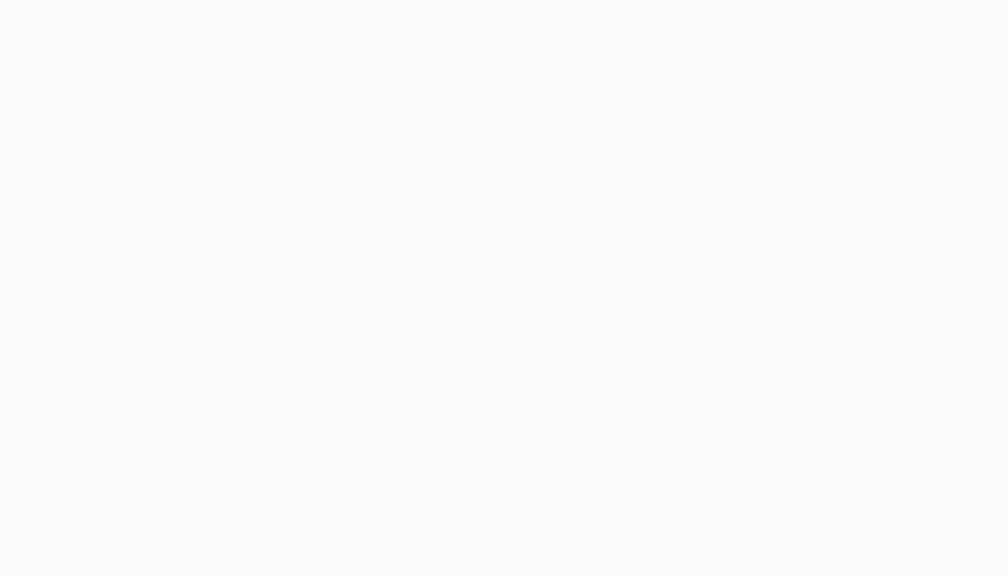All students in JHS are to take this BECE Mathematics Trial Test for JH3 students (Test 1). This test aims at assessing the understanding of candidates as far us JHS2 topics in Mathematics are concerned.

For both section A and B questions, we require students to show working. Answers should be showed to the Mathematics teacher.

Mathematics teachers can also use these questions as revision exercises for JHS2 and JHS3 students.

Let us prepare together for the upcoming BECE as we take the BECE Mathematics Trial Test for JH3 students (Test 1).

ExamHall.org has uploaded several BECE Questions for other subjects already which can be found at HERE

Get more test questions for all subjects HERE

## BECE Mathematics Trial Test for JH3 students (Test 1)

1.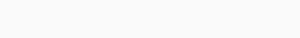X is a point on the line segment AB such that
|AB| = 10cm and |AX| = 4cm. Find the ratio
|AX| : |XB|
A) 5:3
B) 3:2
C) 5:4
D) 2:3

2. If 21:2x = 7:10, find x.
A) 3
B) 2 ¼
C) 15
D) 35

3. Kofi and Ama shared an amount of money in the ratio 3:2 respectively. If Kofi had ¢60,000.00, how much was shared?
A) ¢36,000.00
B) ¢40,000.00
C) ¢90,000.00
D) ¢100,000.00

4. Ten students in Kwamekrom JSS took 9 days to weed the school compound. How long would 15 students take to weed the compound if they worked at the same rate?
A) 5 days
B) 6 days
C) 13 ½ days
D) 14 days

5. 8 girls can weed a plot of land in 10 days. How many days will 5 girls take to weed the same plot of land, working at the same rate?
A) 6 days
B) 8 days
C) 12 days
D) 16 days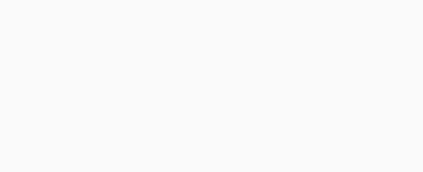6. The figure above is made up of a rectangle and a triangle. The dimensions of the rectangle are 8cm and 6cm. The triangle has 6cm as its base and 3cm as its height. Find the area of the figure.
A) 33cm2
B) 48cm2
C) 51cm2
D) 57cm2

7. PQR is a right-angled triangle. The area of the triangle is 6cm2 and |QR| = 3cm.
Find |PQ|.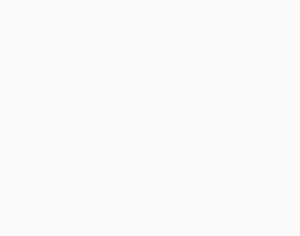3cmA) 2cm
B) 3cm
C) 4cm
D) 6cm

Use the information below to answer questions 8 to 9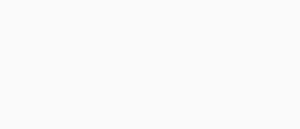In the diagram above, the cylinder has a diameter 4cm and length 14cm. (Take π = 22/7)

8. Find the circumference of the base.
A) 44/7 cm
B) 88/7 cm
C) 176/7 cm
D) 44 cm
9. The area of the base is
A) 176cm2
B) 44cm2
C) 176/7 cm2
D) 88/7 cm2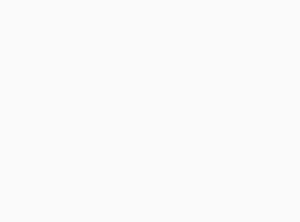10. The area of circle, centre O, is 120cm2. Angle AOB is 60°. Find the area of sector AOB.
A) 2cm2
B) 3cm2
C) 6cm2
D) 20cm2

11. A circle has radius 7cm; find its area. (Take π = 22/7)
A) 22 cm2
B) 44 cm2
C) 145 cm2
D) 154 cm2

12. Find the area of a circle whose diameter is
28 cm.
(Take π = 22/7)
A) 44 cm2
B) 88 cm2
C) 352 cm2
D) 616 cm2

13. Find the circumference of a circle with radius 3.5 cm. (Take π = 22/7)
A) 11 cm
B) 22 cm
C) 35 cm

D) 38.5 cm

The diagram below shows a circle with centre O, S and T are points on the circle. Use it to answer questions 14 and 15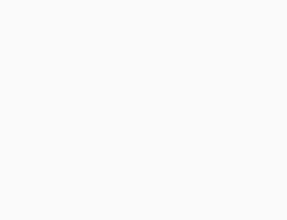14. What name is given to the shaded region ?
A) Sector
B) Segment
D) arc

15. The line ST is called
A) an arc
B) a chord
C) a diameter

16. A square of side 6cm has the same area as a rectangle of length 9cm. Find the breadth of the rectangle.
A) 3cm
B) 4cm
C) 6cm
D) 24cm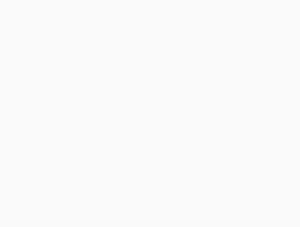17. In the diagram above, A is the centre of the circle with radius 20cm. If angle BAC is 90°, find the perimeter of the shaded sector.
[Take π = 3.14]
A) 31.4cm
B) 31.8cm
C) 40.0cm
D) 51.4cm

18. A table which cost ¢2,400.00 to manufacture was sold for ¢3,000.00. Find the profit percent.
A) 80%
B) 25%
C) 24%
D) 20%
19. Find the simple interest on ¢28,000.00 at 3 ½
% per annum for 6 months.
A) ¢490.00
B) ¢560.00
C) ¢980.00

D) ¢4,000.0020. Kojo paid ¢270,000.00 for a TV set after he had been given a discount of 10%. Find the marked price.

A) ¢300,000.00
B) ¢297,000.00
C) ¢280,000.00
D) ¢260,000.00

## SECTION B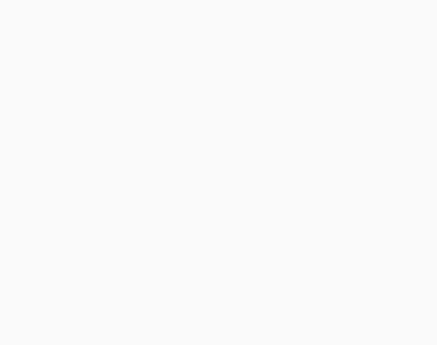The diagram AEBCD shows the shape of Mr. Awuah’s garden, which is made up of a rectangular portion ABCD and a triangular portion AEB.

|AB| = |DC| = 90m, |AD|=|BC|=70m, |AE|=48.5m and |EB|=50m. The height of the triangle is 20m.
(a) Find the
(i) Area of ABCD
(ii) Area of AEB
(iii) Total area of the garden
(iv) Perimeter of the garden.

## One thought on “BECE Mathematics Trial Test for JH3 students (Test 1)”

1.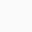Emmanuel says:

Please I need the PDF format